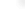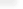Statistical Model Analysis

Taking advantage of Mathematica's full integration of numeric and symbolic mathematics and its complete programming language, Mathematica 7 provides a structured framework for fitting and analyzing linear, nonlinear, and generalized linear models. Built-in functions make it easy to extract, visualize and perform further computations on standard built-in diagnostics and results from fitted models. Together with Mathematica's data import, graphics, and document generation features, Mathematica 7 provides a natural environment for easily fitting, analyzing and generating reports about models of data.

Related Functions